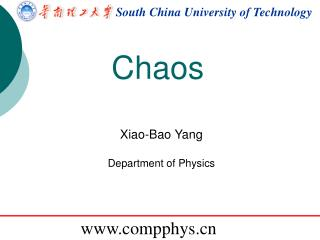DownloadDownload PresentationSouth China University of Technology

# South China University of Technology

Download Presentation## South China University of Technology

- - - - - - - - - - - - - - - - - - - - - - - - - - - E N D - - - - - - - - - - - - - - - - - - - - - - - - - - -
##### Presentation Transcript

1. South China University of Technology Chaos Xiao-BaoYang Department of Physics www.compphys.cn

2. Typically, there are mEqs and m + m(m −1)/2 unknowns. Review of R-K method

3. Decay problem for j=1:nstep; tmp=Nu(j)-Nu(j)/tau*dt/2; Nu(j+1)=Nu(j)-tmp/tau*dt; t(j+1)=t(j)+dt; end

4. Harmonic oscillation for j=1:1000; E(j+1)=.5*m*l^2*(omega(j)^2+g/l*theta(j)^2); d1 = theta(j)+0.5*dt * omega(j); k1 = omega(j)+0.5*dt * (-g/l*theta(j)); theta(j+1)=theta(j)+k1*dt; omega(j+1)=omega(j)+dt*(-g/l*d1); t(j+1)=t(j)+dt; end

5. Non-inertial reference frame

6. Foucault pendulum for ii=1:7000 vx(ii+1)=vx(ii)+(-x(ii)*g/l+2*omega*vy(ii)*sin(fi))*dt; vy(ii+1)=vy(ii)+(-y(ii)*g/l-2*omega*vx(ii)*sin(fi))*dt; x(ii+1)=x(ii)+vx(ii+1)*dt; y(ii+1)=y(ii)+vy(ii+1)*dt; t(ii+1)=t(ii)+dt; end

7. Butterfly Effect

8. A Driven Nonlinear Pendulum Adding dissipation, driving force, and nonlinearity.

9. Chaos in the driven nonlinear pendulum Unpredictable vs. determination Code demonstration ! (Runge Kutta method & Euler-Cromer method)

10. Code l=9.8; g=9.8; q=0.5;FD=1.465;omegaD=2/3; t(1)=0; theta(1)=0.2; omega(1)=0; dt = 0.04; Initialization for i=1:2500; omega(i+1)=omega(i)+dt * (-g/l*(sin(theta(i))) - q * omega(i) + FD*sin(omegaD*t(i))); theta(i+1)=theta(i)+omega(i+1)*dt; t(i+1) = t(i) + dt; %angle transitons end while(theta(i+1)>pi) theta(i+1)=theta(i+1)-2*pi; end while(theta(i+1)<-pi) theta(i+1)=theta(i+1)+2*pi; end plot(t,theta,'k') plot(t,mod(theta+pi,2*pi)-pi,'k')

11. Everything predictable? • 洛倫茨……結束了笛卡爾宇宙觀統治的時代，繼相對論和量子力學之後，開啟了20世紀第三次科學革命。”——麻省理工學院大氣學教授伊曼紐爾 •     “他的混沌理論繼牛頓之後，為人類自然觀帶來了最為戲劇性的改變。” • ——1991年“京都獎”評委會致辭

12. Chaos in the driven nonlinear pendulum Illustration!

13. clear l=9.8; g=9.8; q=0.5;FD=0.5;omegaD=2/3; t(1)=0; theta(1)=0.2; omega(1)=0; dt = 0.04; for i=1:1500; omega(i+1)=omega(i)+dt * (-g/l*(sin(theta(i))) - q * omega(i) + FD*sin(omegaD*t(i))); theta(i+1)=theta(i)+omega(i+1)*dt; t(i+1) = t(i) + dt; end code Initialization Calculation Little change Calculation Show difference tmp=theta; theta(1)=0.2+0.001; plot(t,log(abs(tmp-theta))/log(10))

14. Lyapunov Exponent In mathematics, the Lyapunov exponent or Lyapunov characteristic exponent of a dynamical system is a quantity that characterizes the rate of separation of infinitesimally close trajectories. Lyapunov (1857-1918) is known for his development of the stability theory of a dynamical system, as well as for his many contributions to mathematical physics and probability theory.

15. Chaos in the driven nonlinear pendulum Illustration!

16. Jules Henri Poincaré (1854─1912) In mathematics, particularly in dynamical systems, a first recurrence map or Poincaré map, named after Henri Poincaré, is the intersection of a periodic orbit in the state space of a continuous dynamical system with a certain lower dimensional subspace, called the Poincaré section, transversal to the flow of the system. In mathematics, the Poincaré recurrence theorem states that certain systems will, after a sufficiently long time, return to a state very close to the initial state. The Poincaré recurrence time is the length of time elapsed until the recurrence. The result applies to physical systems in which energy is conserved. The theorem is commonly discussed in the context of ergodic theory, dynamical systems and statistical mechanics. The theorem is named after Henri Poincaré, published in 1890.

17. Chaos in the driven nonlinear pendulum

18. Code Initialization Calculation Data selecting clear l=9.8; g=9.8; q=0.5;FD=0.5;omegaD=2/3; t(1)=0; theta(1)=0.2; omega(1)=0; dt = 0.04; for i=1:20000; omega(i+1)=omega(i)+dt * (-g/l*(sin(theta(i))) - q * omega(i) + FD*sin(omegaD*t(i))); theta(i+1)=theta(i)+omega(i+1)*dt; t(i+1) = t(i) + dt; end re=[]; for ii=500:length(t)-1 if cos(t(ii)*omegaD)>cos(t(ii-1)*omegaD))&(cos(t(ii)*omegaD)>cos(t(ii+1)*omegaD)) re=[re ii]; end end theta=mod(theta+pi,2*pi)-pi; plot(theta(re),omega(re),'.')

19. Routes to Chaos: Period Doubling

20. Routes to Chaos: Period Doubling Only θ at t in phase of FD, i.e. (observe θ at a particular time in the drive cycle) Here Feigenbaum constant

21. The Logistic Map: Why The Period Doubles Population growth model in a collection of animals: Illustration!

22. The Logistic Map: Why The Period Doubles

23. The Logistic Map: Why The Period Doubles

24. The Lorentz Model Lorentz model (Simplified Navier-Stokes equations) Numerical solution

25. The Lorentz Model

26. The Lorentz Model

27. The Lorentz Model

28. Fixed point for solving equations(P469) Root finding Newton-Raphson method secant method

29. Homework • Exercise: • 3.9, 3.12, 3.23 • Both Results and source codes are required.